Like   Tweet
 /* styles */ June 10, 2020 As we watch the world unfold and refold itself daily and strive to understand ourselves and each other, the Finalists of the Dayton Literary Peace Prize have contributed insightful books about racial issues to help us in that mission. We recommend these books to you.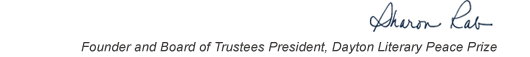table div table+table+table+table div table{width:100%;padding:0}table div table+table+table+table div table img{width:96.23%;padding:0;float:none}table div table+table+table+table div table td{width:100%;padding:0 1.88% 18px}/* styles *//* styles */ “Read global; buy local.” — Marlon James, DLPP 2009 Fiction Winner for The Book of Night Women
 table div table+table+table+table+table+table div table{width:100%;padding:0}table div table+table+table+table+table+table div table img{width:96.23%;padding:0;float:none}table div table+table+table+table+table+table div table td{width:100%;padding:0 1.88% 18px}/* styles */## Finalists Who Give Insight on Race: The Books

 table div table+table+table+table+table+table+table+table div table{width:100%;padding:0}table div table+table+table+table+table+table+table+table div table img{width:96.23%;padding:0;float:none}table div table+table+table+table+table+table+table+table div table td{width:100%;padding:0 1.88% 18px}/* styles */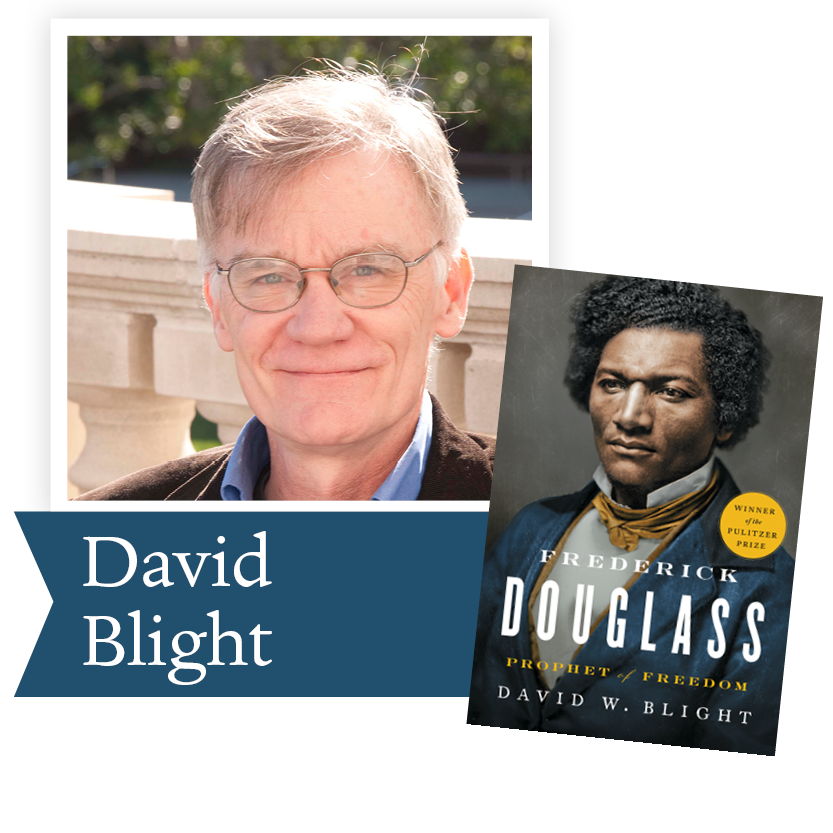2019, Frederick Douglass by David Blight (Simon and Schuster): In his “cinematic and deeply engaging” (The New York Times Book Review) biography, Blight has drawn on new information held in a private collection that few other historians have consulted, as well as recently discovered issues of Douglass’s newspapers. Blight’s Pulitzer Prize-winning book tells the fascinating story of Douglass’s two marriages and his complex extended family.

 table div table+table+table+table+table+table+table+table+table+table div table{width:100%;padding:0}table div table+table+table+table+table+table+table+table+table+table div table img{width:96.23%;padding:0;float:none}table div table+table+table+table+table+table+table+table+table+table div table td{width:100%;padding:0 1.88% 18px}/* styles */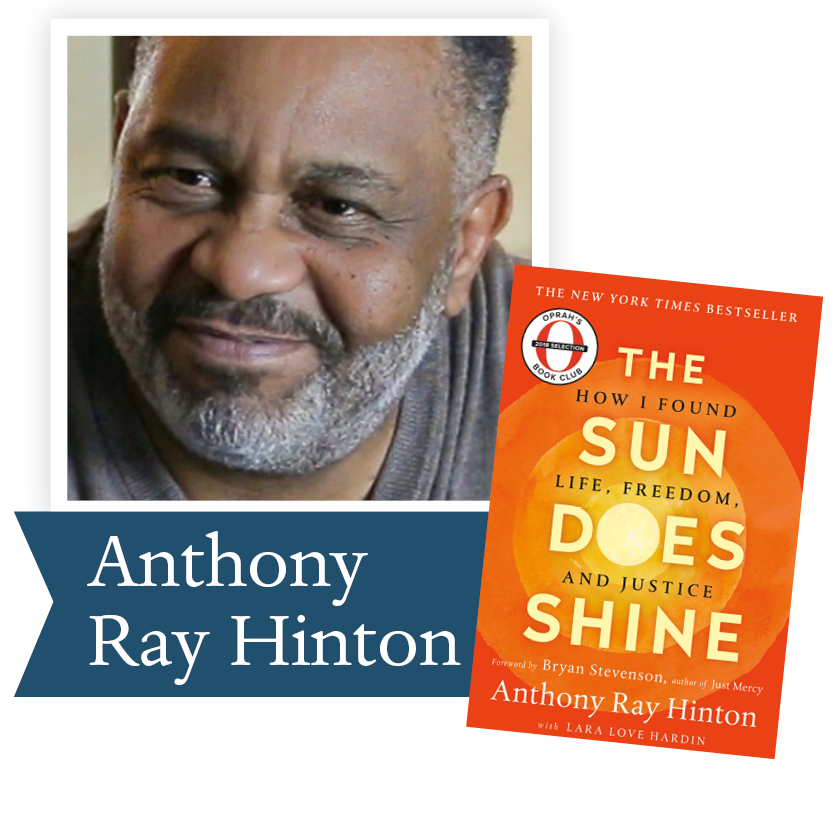2019, The Sun Does Shine: How I Found Life and Freedom on Death Row by Anthony Ray Hinton with Laura Love Hardin (St. Martins): With a foreword by Bryan Stevenson, The Sun Does Shine is an extraordinary testament to the power of hope sustained through the darkest times. Destined to be a classic memoir of wrongful imprisonment and freedom won, The Sun Does Shine tells Hinton’s dramatic 30-year journey and shows how you can take away a man’s freedom, but you can’t take away his imagination, humor, or joy.

 table div table+table+table+table+table+table+table+table+table+table+table+table div table{width:100%;padding:0}table div table+table+table+table+table+table+table+table+table+table+table+table div table img{width:96.23%;padding:0;float:none}table div table+table+table+table+table+table+table+table+table+table+table+table div table td{width:100%;padding:0 1.88% 18px}/* styles */2018, Sing, Unburied, Sing by Jesmyn Ward (Scribner): Following a family making the trip from their Gulf Coast town to the Mississippi State Penitentiary, testing the strength of emotional bonds and the pull of our collective history.

 table div table+table+table+table+table+table+table+table+table+table+table+table+table+table div table{width:100%;padding:0}table div table+table+table+table+table+table+table+table+table+table+table+table+table+table div table img{width:96.23%;padding:0;float:none}table div table+table+table+table+table+table+table+table+table+table+table+table+table+table div table td{width:100%;padding:0 1.88% 18px}/* styles */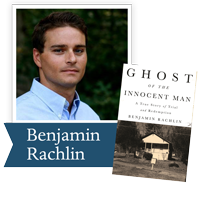2018, Ghost of the Innocent Man by Benjamin Rachlin (Little, Brown): A gripping account of one man's long road to freedom that will forever change how we understand our criminal justice system through one of the most dramatic of those cases. It provides a picture of wrongful conviction and of the opportunity for meaningful reform.

 table div table+table+table+table+table+table+table+table+table+table+table+table+table+table+table+table div table{width:100%;padding:0}table div table+table+table+table+table+table+table+table+table+table+table+table+table+table+table+table div table img{width:96.23%;padding:0;float:none}table div table+table+table+table+table+table+table+table+table+table+table+table+table+table+table+table div table td{width:100%;padding:0 1.88% 18px}/* styles */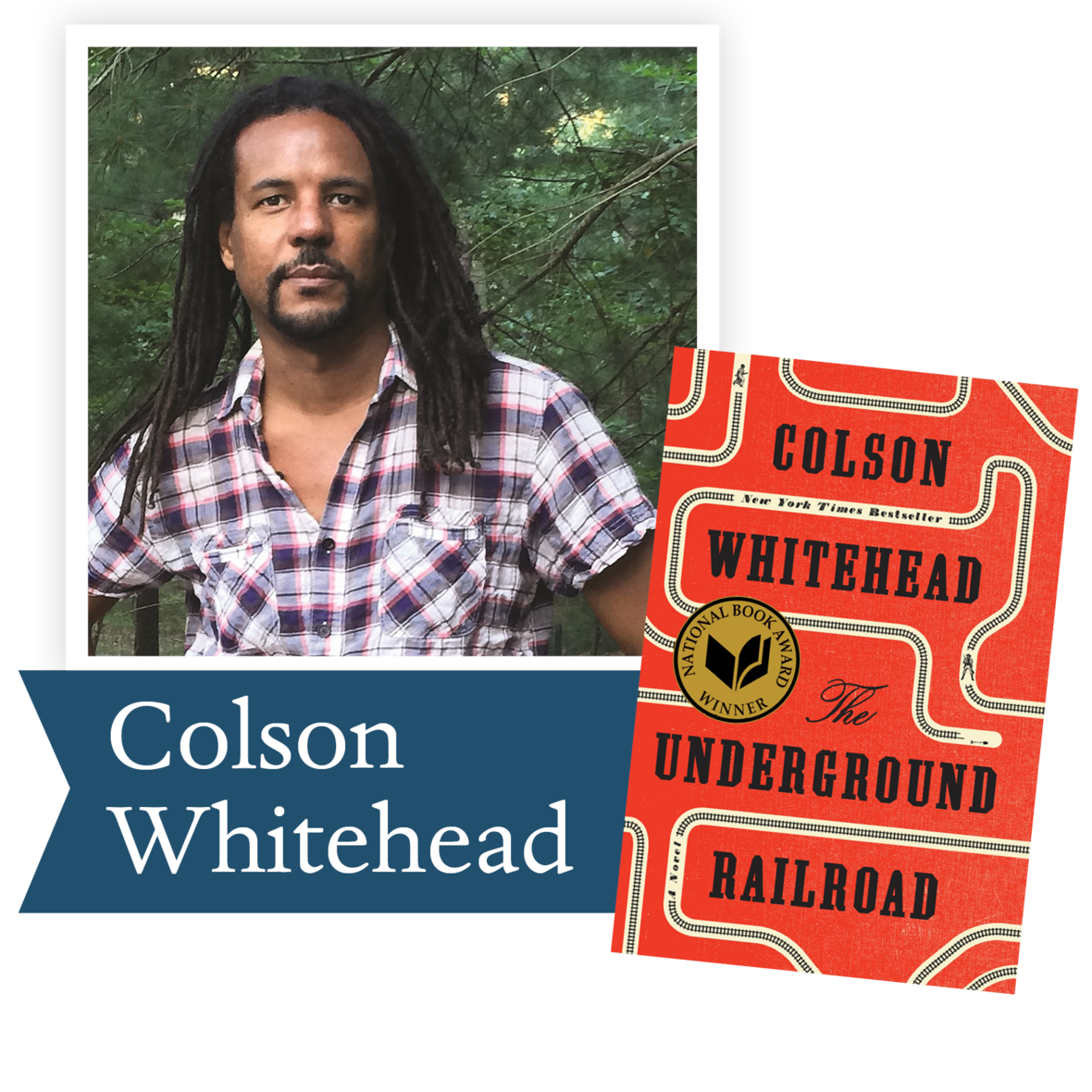2017, The Underground Railroad by Colson Whitehead (Doubleday): Winner of the Pulitzer Prize and the National Book Award, the #1 New York Times bestseller from Colson Whitehead, a magnificent tour de force chronicling a young slave's adventures as she makes a desperate bid for freedom in the antebellum South.

 table div table+table+table+table+table+table+table+table+table+table+table+table+table+table+table+table+table+table div table{width:100%;padding:0}table div table+table+table+table+table+table+table+table+table+table+table+table+table+table+table+table+table+table div table img{width:96.23%;padding:0;float:none}table div table+table+table+table+table+table+table+table+table+table+table+table+table+table+table+table+table+table div table td{width:100%;padding:0 1.88% 18px}/* styles */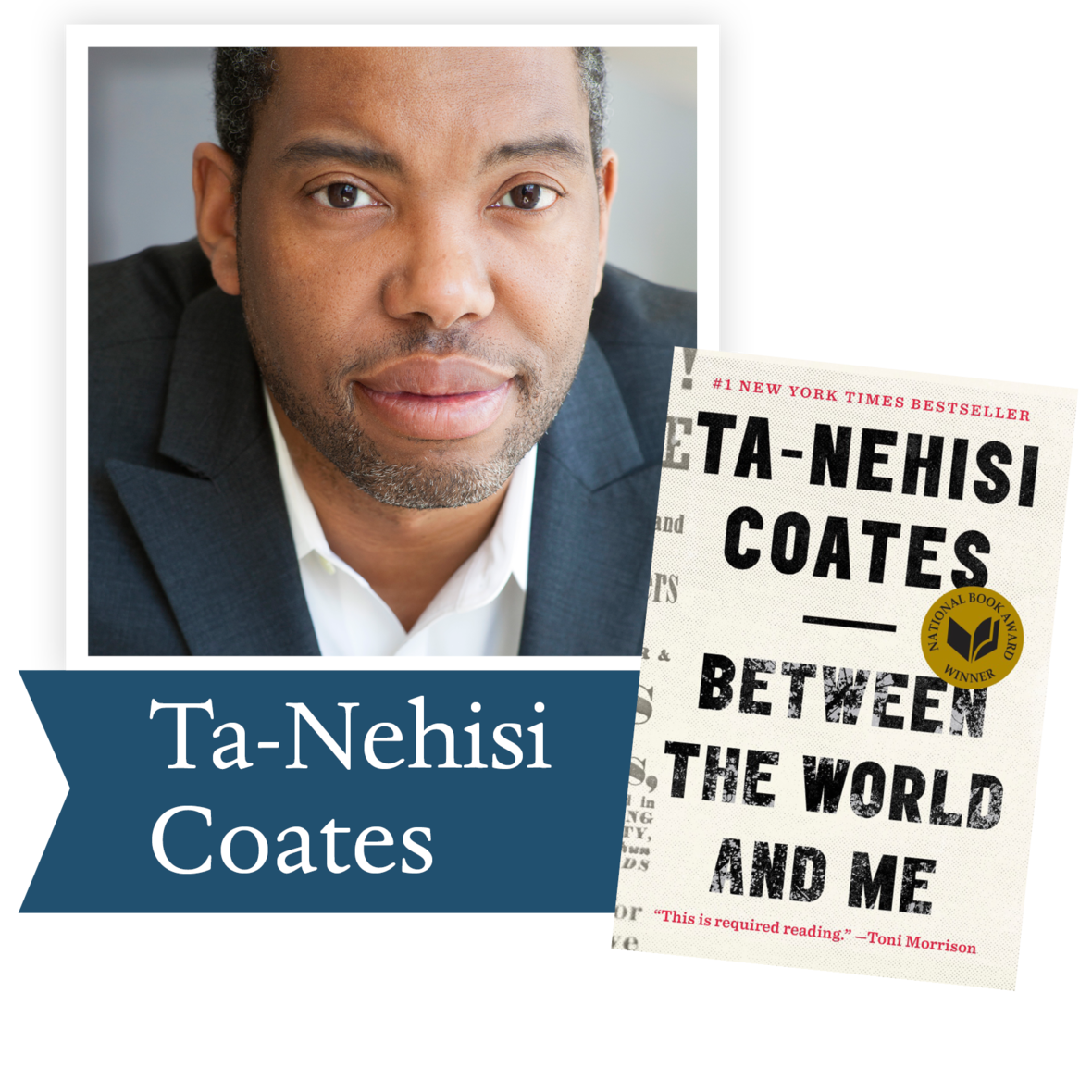2016, Between the World and Me by Ta-Nehisi Coates (Spiegel & Grau): The author shares with his son—and readers—the story of his own awakening to the truth about history and his place in the world through a series of revelatory experiences. Taken together, these stories map a journey from fear and confusion to a full understanding of this country, this world and the pursuit of freedom.

 table div table+table+table+table+table+table+table+table+table+table+table+table+table+table+table+table+table+table+table+table div table{width:100%;padding:0}table div table+table+table+table+table+table+table+table+table+table+table+table+table+table+table+table+table+table+table+table div table img{width:96.23%;padding:0;float:none}table div table+table+table+table+table+table+table+table+table+table+table+table+table+table+table+table+table+table+table+table div table td{width:100%;padding:0 1.88% 18px}/* styles */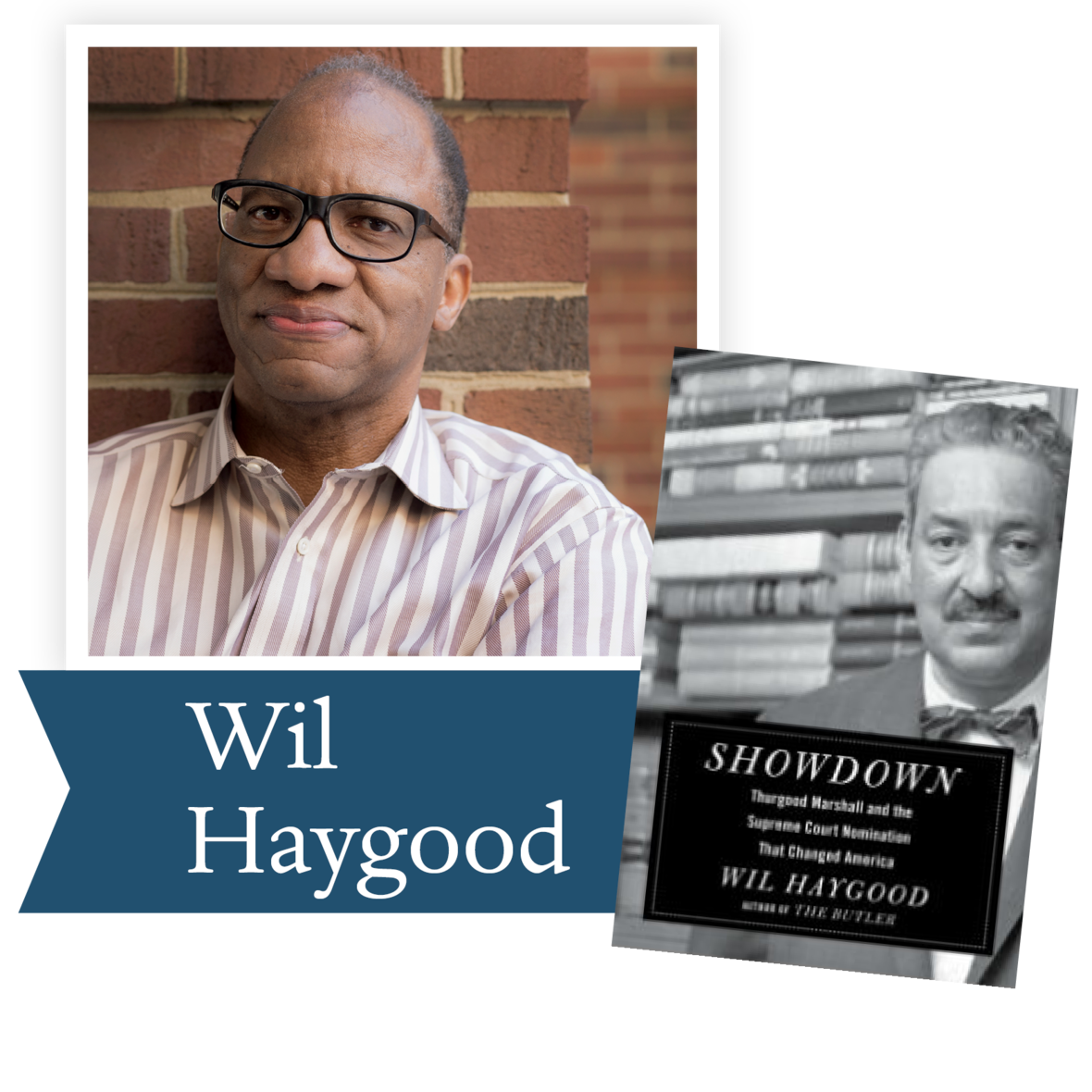2016, Showdown by Wil Haygood (Knopf): The author details the life of Thurgood Marshall, the first African-American Supreme Court justice and one of the most transformative legal minds of the past one hundred years. Haygood creates a provocative look at Marshall’s life and the politicians, lawyers, activists, and others who shaped—or desperately tried to stop—the Civil Rights Movement.

 table div table+table+table+table+table+table+table+table+table+table+table+table+table+table+table+table+table+table+table+table+table+table div table{width:100%;padding:0}table div table+table+table+table+table+table+table+table+table+table+table+table+table+table+table+table+table+table+table+table+table+table div table img{width:96.23%;padding:0;float:none}table div table+table+table+table+table+table+table+table+table+table+table+table+table+table+table+table+table+table+table+table+table+table div table td{width:100%;padding:0 1.88% 18px}/* styles */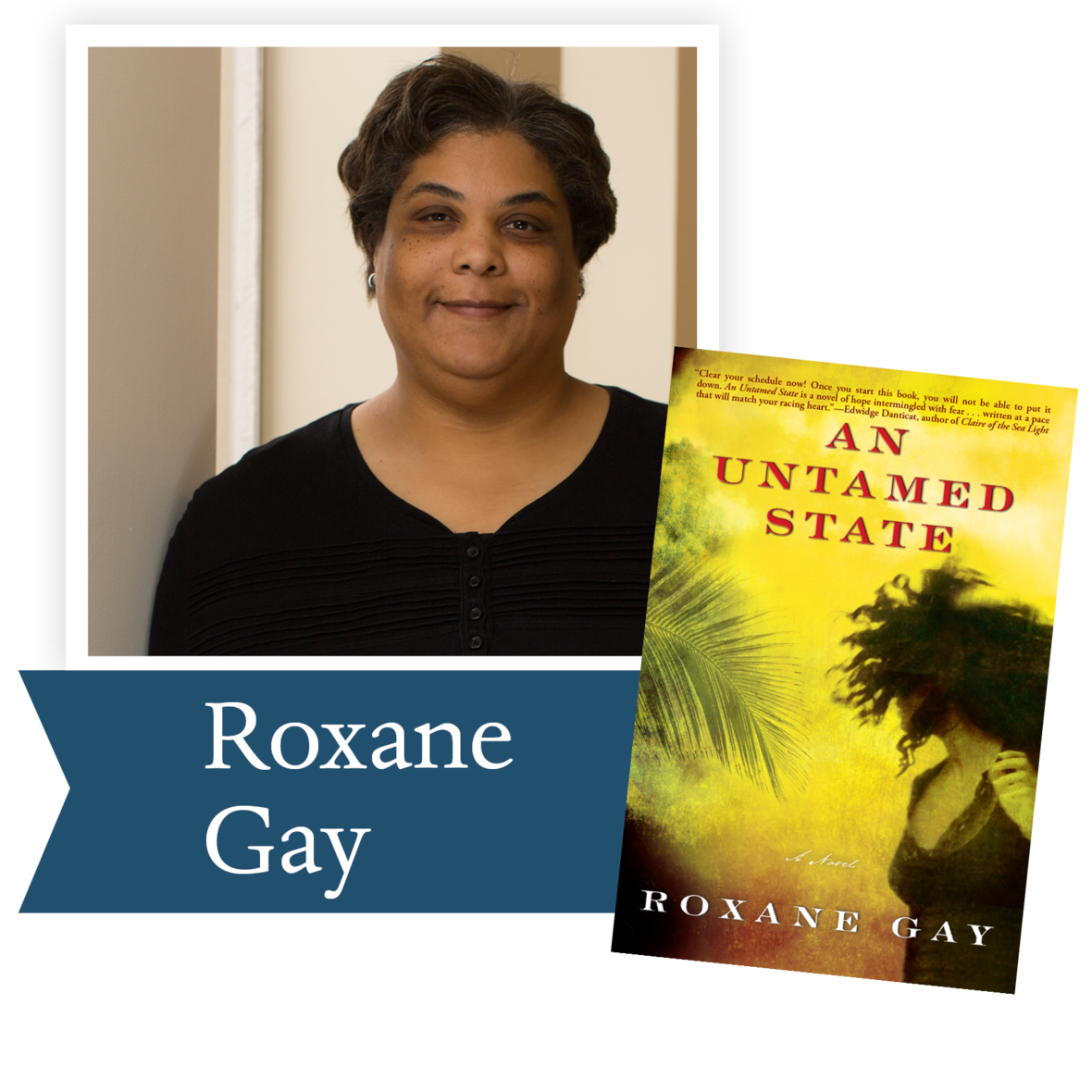2015, An Untamed State by Roxane Gay (Grove Atlantic): Roxane Gay delivers an assured debut with a story of privilege in the face of crushing poverty, and of the lawless
anger that corrupt governments produce, finally showing us how redemption is found in the most unexpected of places.

 table div table+table+table+table+table+table+table+table+table+table+table+table+table+table+table+table+table+table+table+table+table+table+table+table div table{width:100%;padding:0}table div table+table+table+table+table+table+table+table+table+table+table+table+table+table+table+table+table+table+table+table+table+table+table+table div table img{width:96.23%;padding:0;float:none}table div table+table+table+table+table+table+table+table+table+table+table+table+table+table+table+table+table+table+table+table+table+table+table+table div table td{width:100%;padding:0 1.88% 18px}/* styles */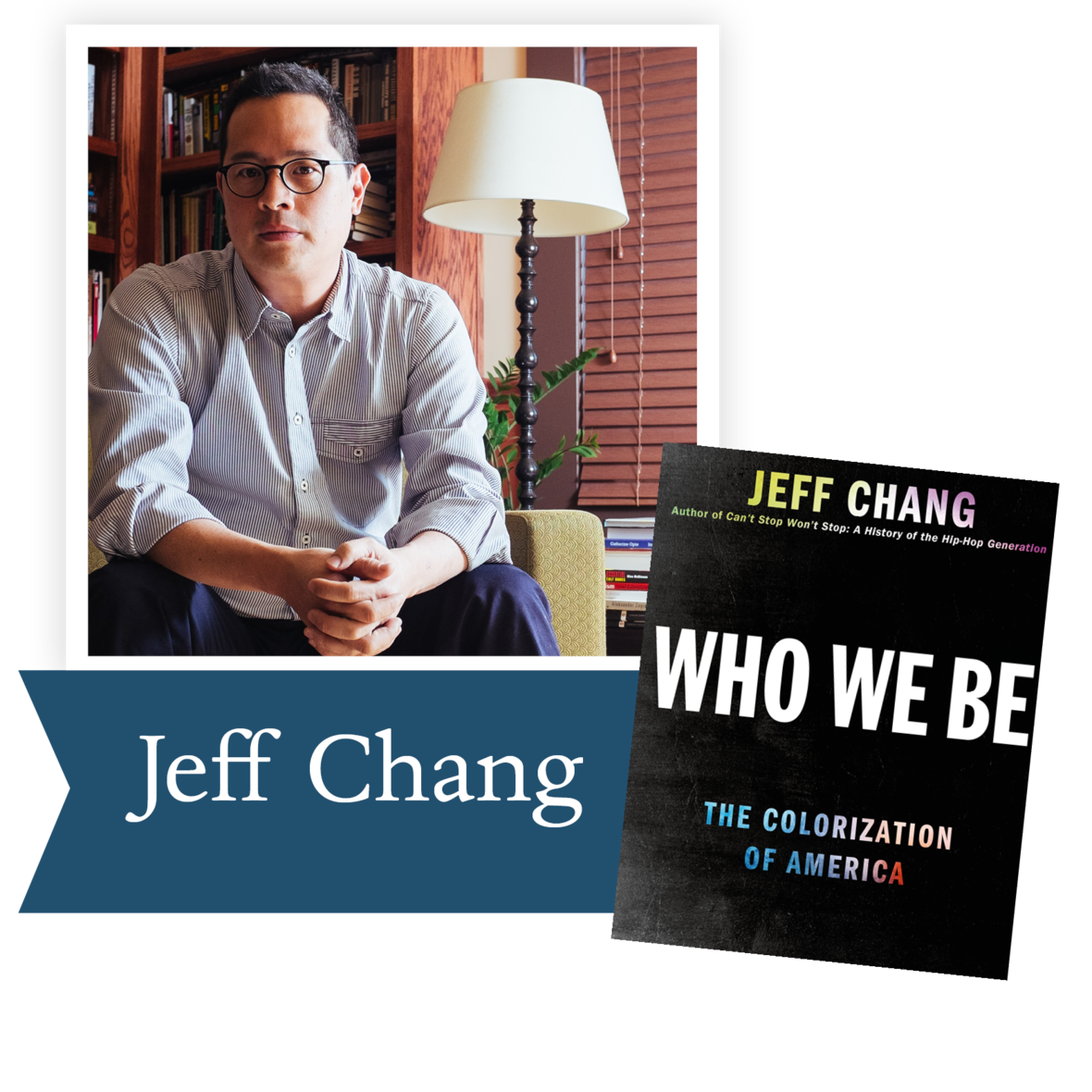2015, Who We Be by Jeff Chang (St. Martin’s Press): This book explores the changing (and unchanging) ways that the U.S. has viewed race over the past half-century, asking whether or not, in the eras of “multicultural” and “post-racial” cultures, we really see each other more clearly.

 table div table+table+table+table+table+table+table+table+table+table+table+table+table+table+table+table+table+table+table+table+table+table+table+table+table+table div table{width:100%;padding:0}table div table+table+table+table+table+table+table+table+table+table+table+table+table+table+table+table+table+table+table+table+table+table+table+table+table+table div table img{width:96.23%;padding:0;float:none}table div table+table+table+table+table+table+table+table+table+table+table+table+table+table+table+table+table+table+table+table+table+table+table+table+table+table div table td{width:100%;padding:0 1.88% 18px}/* styles */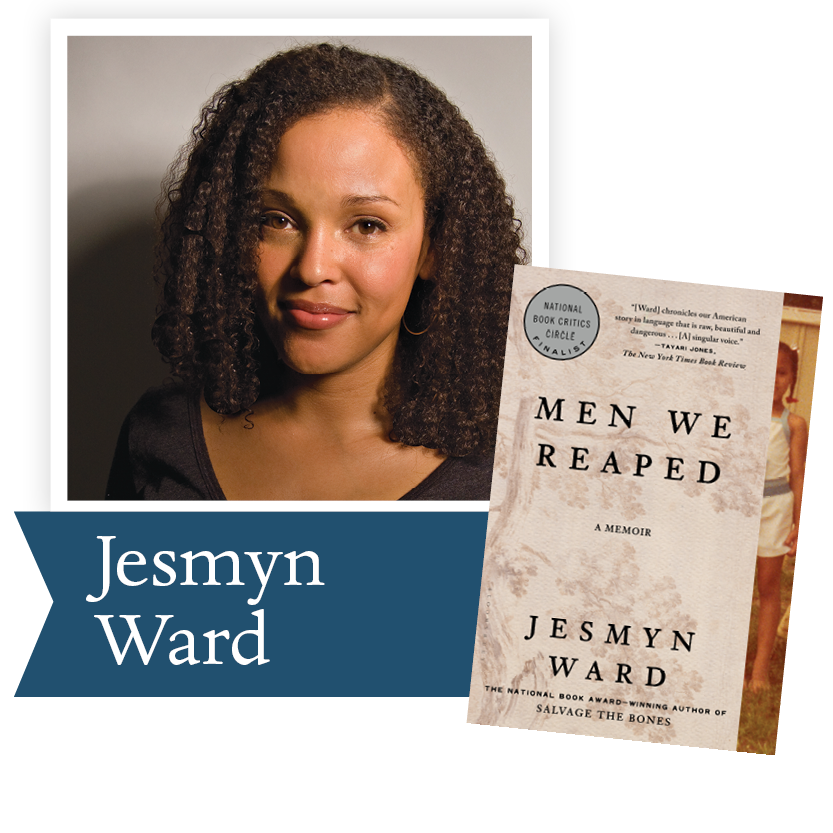2014, Men We Reaped: A Memoir by Jesmyn Ward (Bloomsbury): In this universally acclaimed memoir, Ward recounts the separate deaths of five young men—all dear to her—from her small Mississippi community, agonizingly tracing each one back to the long-term effects of racism and economic disadvantage.

 table div table+table+table+table+table+table+table+table+table+table+table+table+table+table+table+table+table+table+table+table+table+table+table+table+table+table+table+table div table{width:100%;padding:0}table div table+table+table+table+table+table+table+table+table+table+table+table+table+table+table+table+table+table+table+table+table+table+table+table+table+table+table+table div table img{width:96.23%;padding:0;float:none}table div table+table+table+table+table+table+table+table+table+table+table+table+table+table+table+table+table+table+table+table+table+table+table+table+table+table+table+table div table td{width:100%;padding:0 1.88% 18px}/* styles */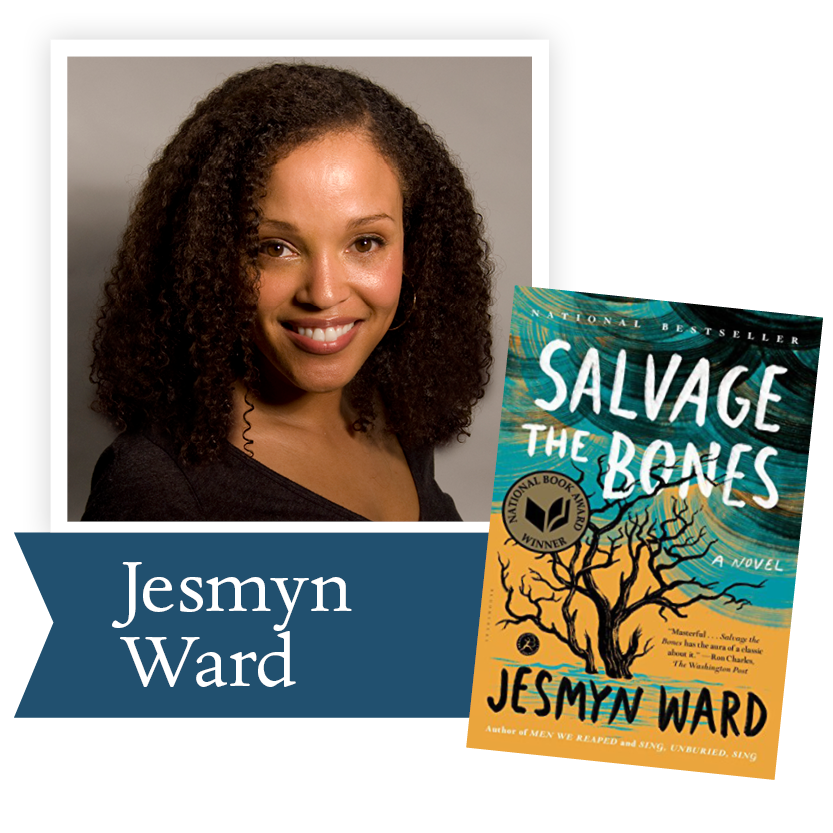2012, Salvage the Bones by Jesmyn Ward
(Bloomsbury): A big-hearted novel about familial love and community against all odds and a wrenching look at the lonesome, brutal, and restrictive realities of rural poverty.

 table div table+table+table+table+table+table+table+table+table+table+table+table+table+table+table+table+table+table+table+table+table+table+table+table+table+table+table+table+table+table div table{width:100%;padding:0}table div table+table+table+table+table+table+table+table+table+table+table+table+table+table+table+table+table+table+table+table+table+table+table+table+table+table+table+table+table+table div table img{width:96.23%;padding:0;float:none}table div table+table+table+table+table+table+table+table+table+table+table+table+table+table+table+table+table+table+table+table+table+table+table+table+table+table+table+table+table+table div table td{width:100%;padding:0 1.88% 18px}/* styles */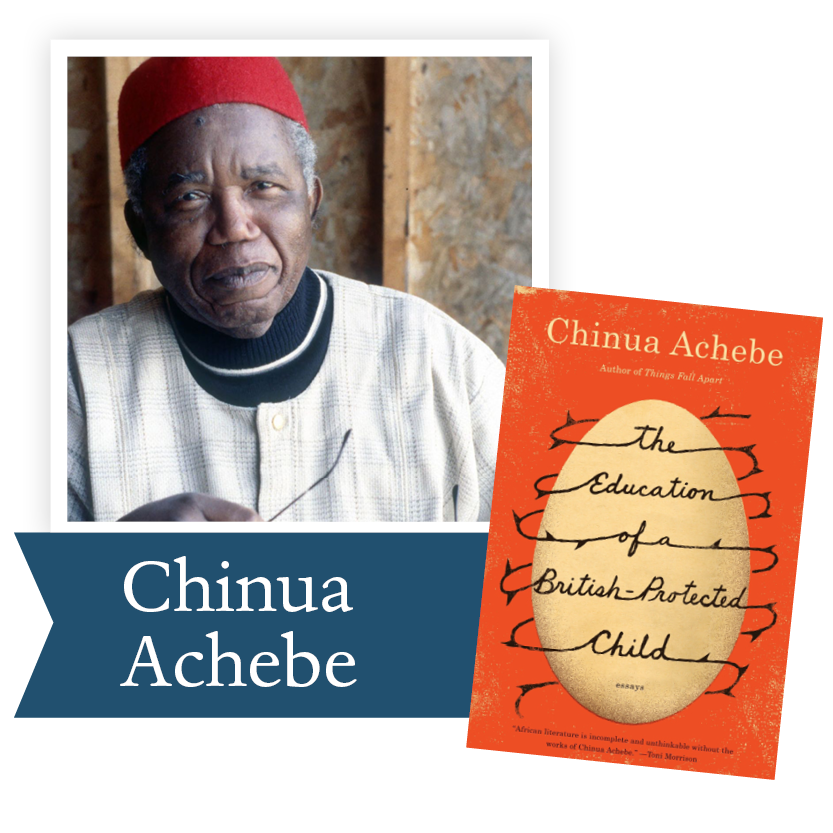2010, The Education of a British-Protected Child by Chinua Achebe (Knopf): From the celebrated author of Things Fall Apart, a new collection of autobiographical essays—his first new book in more than twenty years.

 table div table+table+table+table+table+table+table+table+table+table+table+table+table+table+table+table+table+table+table+table+table+table+table+table+table+table+table+table+table+table+table+table div table{width:100%;padding:0}table div table+table+table+table+table+table+table+table+table+table+table+table+table+table+table+table+table+table+table+table+table+table+table+table+table+table+table+table+table+table+table+table div table img{width:96.23%;padding:0;float:none}table div table+table+table+table+table+table+table+table+table+table+table+table+table+table+table+table+table+table+table+table+table+table+table+table+table+table+table+table+table+table+table+table div table td{width:100%;padding:0 1.88% 18px}/* styles */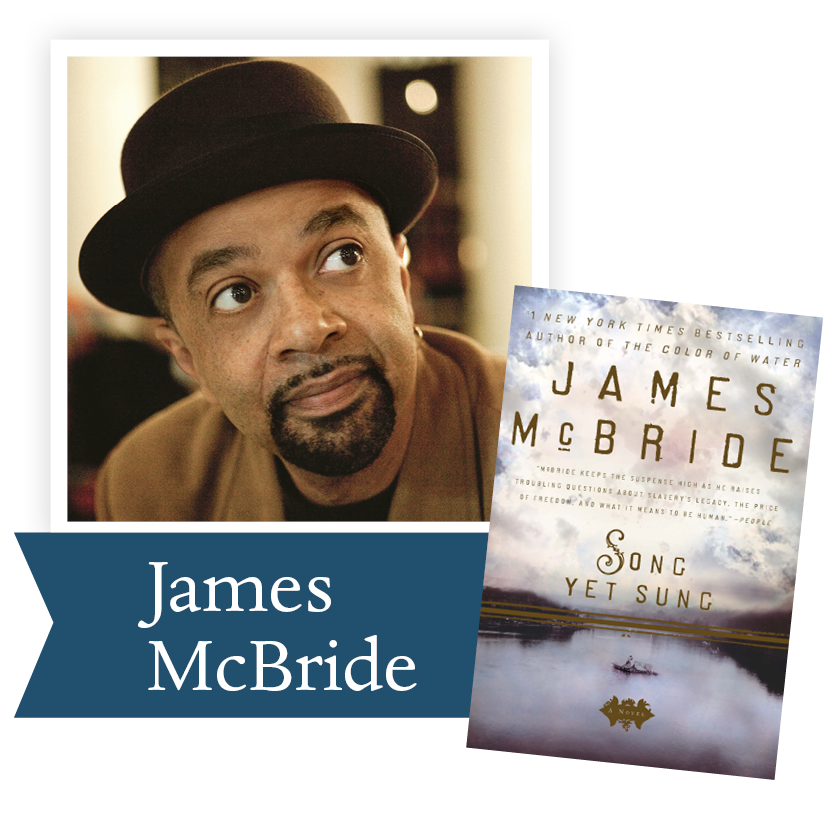2009, Song Yet Sung by James McBride (Penguin Group): The haunting story of a runaway slave and a determined slave-catcher in pre-Civil War Maryland, Song Yet Sung explores both the moral choices faced by both blacks and whites and the meaning of freedom.

 table div table+table+table+table+table+table+table+table+table+table+table+table+table+table+table+table+table+table+table+table+table+table+table+table+table+table+table+table+table+table+table+table+table+table div table{width:100%;padding:0}table div table+table+table+table+table+table+table+table+table+table+table+table+table+table+table+table+table+table+table+table+table+table+table+table+table+table+table+table+table+table+table+table+table+table div table img{width:96.23%;padding:0;float:none}table div table+table+table+table+table+table+table+table+table+table+table+table+table+table+table+table+table+table+table+table+table+table+table+table+table+table+table+table+table+table+table+table+table+table div table td{width:100%;padding:0 1.88% 18px}/* styles */table div table+table+table+table+table+table+table+table+table+table+table+table+table+table+table+table+table+table+table+table+table+table+table+table+table+table+table+table+table+table+table+table+table+table+table+table div table{width:100%;padding:0}table div table+table+table+table+table+table+table+table+table+table+table+table+table+table+table+table+table+table+table+table+table+table+table+table+table+table+table+table+table+table+table+table+table+table+table+table div table img{width:96.23%;padding:0;float:none}table div table+table+table+table+table+table+table+table+table+table+table+table+table+table+table+table+table+table+table+table+table+table+table+table+table+table+table+table+table+table+table+table+table+table+table+table div table td{width:100%;padding:0 1.88% 18px}/* styles */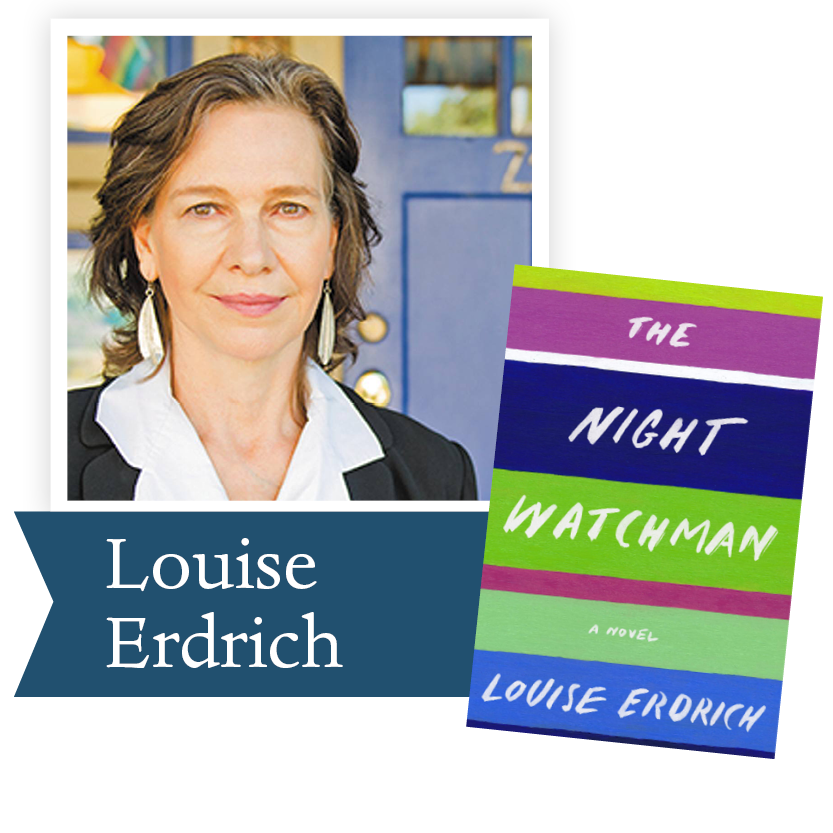New York Times Bestseller

Based on the extraordinary life of National Book Award-winning author Louise Erdrich’s grandfather who worked as a night watchman and carried the fight against Native dispossession from rural North Dakota all the way to Washington, D.C., this powerful novel explores themes of love and death with lightness and gravity and unfolds with the elegant prose, sly humor, and depth of feeling of a master craftsman.

Ron Rollins, newly retired Dayton Daily News Ideas and Voices Editor, will moderate the discussion. Congratulations, Ron!

Our next book club will take place via Zoom on Wednesday, June 17 at 7:00 p.m. Email Emily Kretzer to be added to the list.

 table div table+table+table+table+table+table+table+table+table+table+table+table+table+table+table+table+table+table+table+table+table+table+table+table+table+table+table+table+table+table+table+table+table+table+table+table+table+table div table{width:100%;padding:0}table div table+table+table+table+table+table+table+table+table+table+table+table+table+table+table+table+table+table+table+table+table+table+table+table+table+table+table+table+table+table+table+table+table+table+table+table+table+table div table img{width:96.23%;padding:0;float:none}table div table+table+table+table+table+table+table+table+table+table+table+table+table+table+table+table+table+table+table+table+table+table+table+table+table+table+table+table+table+table+table+table+table+table+table+table+table+table div table td{width:100%;padding:0 1.88% 18px}/* styles */Support the Dayton Literary Peace Prize when you shop with Amazon using this link.